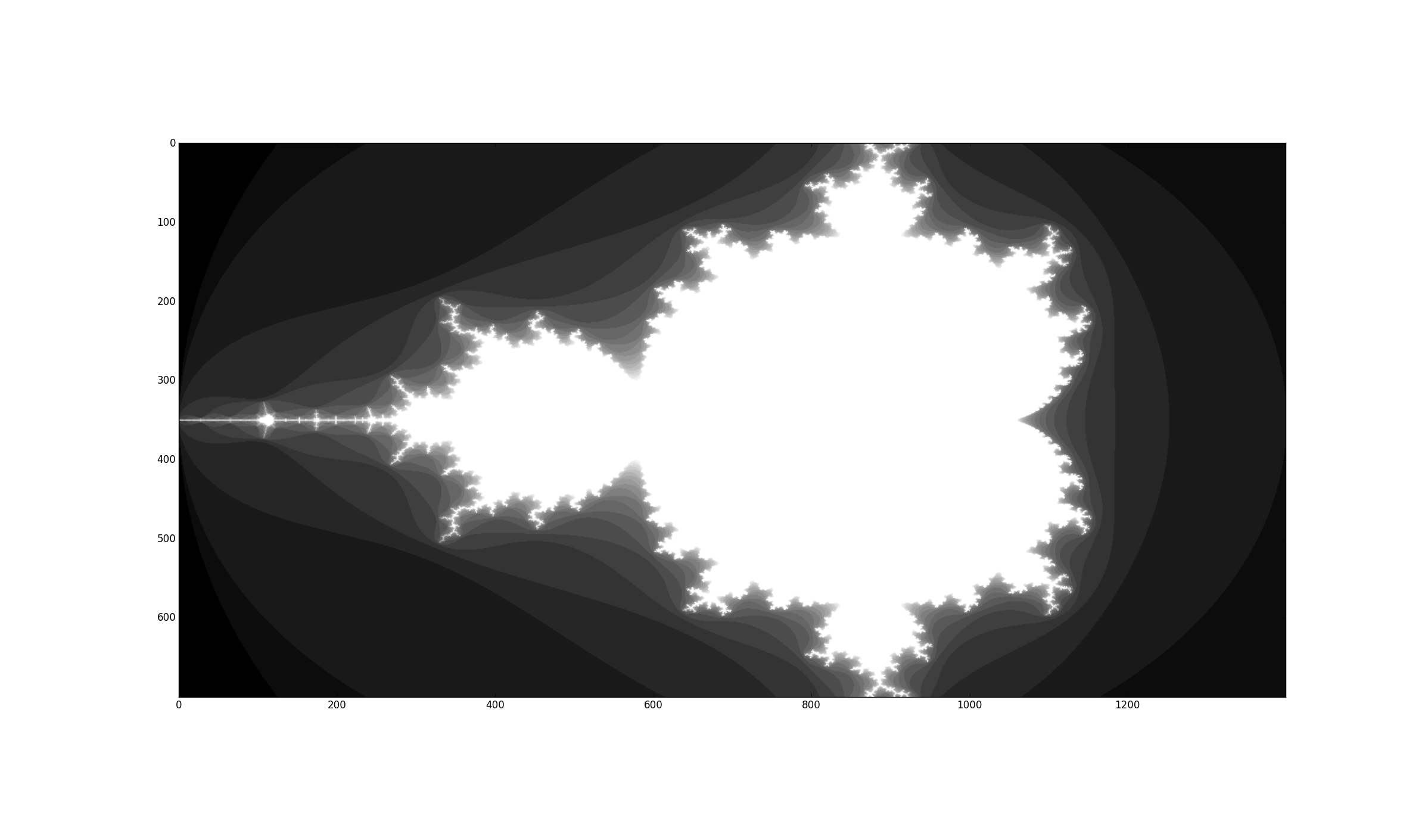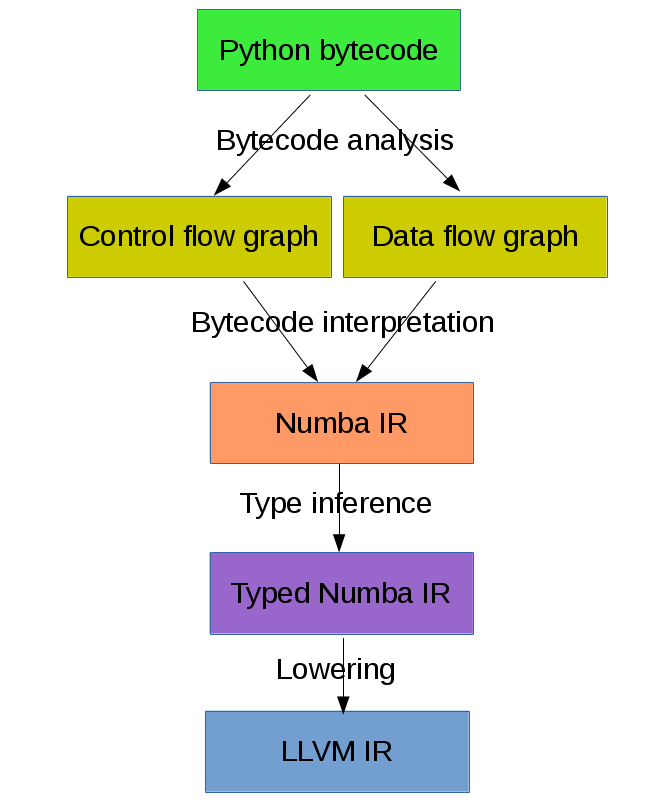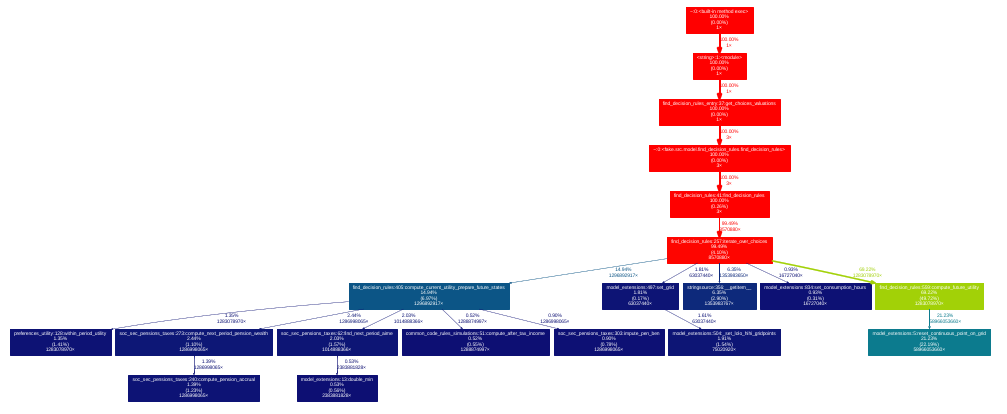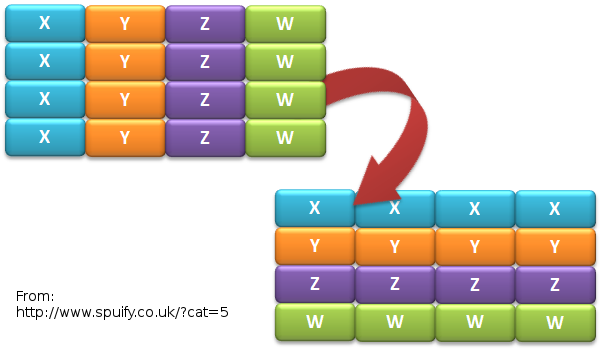# Accelerating Python code with Numba and LLVM

Graham Markall

Compiler Engineer, Embecosm

graham.markall@embecosm.com

1

## Overview

My background:

• Now: Compiler Engineer at Embecosm - GNU Toolchains

• Previously: Engineer at Continuum Analytics
• Numba user, and Numba developer
• Background in Python libraries for HPC (PyOP2, Firedrake)

This talk is an overview of:

• Numba: a Python compiler focused on numerical code
• llvmlite: a lightweight LLVM Python binding for writing JIT compilers
2

## What is Numba? (1)

A tool that makes Python code go faster by specialising and compiling it.

• Mainly focused on array-oriented and numerical code
• Heavily object-oriented, dynamic code not the target use case
• Alternative to using native code (e.g. C-API or CFFI) with C, Fortran, or Cython.
• Keeping all code as Python code:
• Allows focus on algorithmic development
• Minimise development time
3

## What is Numba? (2)

• It's opt-in: Numba only compiles the functions you specify
• Not a whole-program compiler like PyPy or V8
• Not a tracing JIT - always compiles before execution
• Trade off: relaxing the semantics of Python code in return for performance.
• Deliberate narrow focus to handle CPU and non-CPU targets in reasonable way.
4

## Implementation overview

• JIT compiler for Python based on LLVM
• Targets CPUs, CUDA GPUs, HSA APUs
• CPython 2.7, 3.4, 3.5
• Runs side-by-side with Numpy, Scipy, etc ecosystem
• Linux / OS X / Windows
5

## Who uses Numba?

• Scientists, engineers, anyone interested in numerical modelling

Random selection of users:

6

## Numba example

```# Mandelbrot function in Python

def mandel(x, y, max_iters):
c = complex(x,y)
z = 0j
for i in range(max_iters):
z = z*z + c
if z.real * z.real + z.imag * z.imag >= 4:
return 255 * i // max_iters

return 255
```
7

## Numba example

```# Mandelbrot function in Python using Numba
from numba import jit

@jit
def mandel(x, y, max_iters):
c = complex(x,y)
z = 0j
for i in range(max_iters):
z = z*z + c
if z.real * z.real + z.imag * z.imag >= 4:
return 255 * i // max_iters

return 255
```
8

## Mandelbrot, 20 iterations

 CPython 1x Numpy array-wide operations 13x Numba (CPU) 120x Numba (NVidia Tesla K20c) 2100x9

## Other examples

Times in msec:

Example CPython Numba Speedup
Black-Scholes 969 433 2.2x
Check Neighbours 550 28 19.9x
IS Distance 372 70 5.4x
Pairwise 62 12 5.1x
10

## Dispatch process

Calling a `@jit` function:

1. Lookup types of arguments
2. Do any compiled versions match the types of these arguments?
1. Yes: retrieve the compiled code from the cache
2. No: compile a new specialisation
1. Marshal arguments to native values
2. Call the native code function
3. Marshal the native return value to a Python value
11

```@jit
return a + b

return a + b
```
```>>> %timeit add(1, 2)
10000000 loops, best of 3: 163 ns per loop

10000000 loops, best of 3: 85.3 ns per loop
```
12

## Compilation pipeline13

## Type Inference

• Native code is statically typed, Python is not
• Numba contains mappings of input to output types, e.g.:
```float32 + float32 -> float32
int32   + float32 -> float64
```
• Propagates type information using the data flow graph
```def f(a, b):   # a:= float32, b:= float32
c = a + b  # c:= float32
return c   # return := float32
```
14

## Type Unification

Example typing 1:

```def select(a, b, c):  # a := float32, b := float32, c := bool
if c:
ret = a       # ret := float32
else:
ret = b       # ret := float32
return ret       # return := {float32, float32}
#           => float32
```
15

## Type Unification

Example typing 2:

```def select(a, b, c):  # a := tuple(int32, int32), b := float32,
# c := bool
if c:
ret = a       # ret := tuple(int32, int32)
else:
ret = b       # ret := float32
return ret       # return := {tuple(int32, int32), float32}
#           => XXX
```
16

# LLVM Interface

17

## LLVM-PY

• Early versions of Numba used LLVMPY
• Supported LLVM 3.2 / 3.3 using a C++ interface
• Downsides:
• Errors hard to understand (e.g. segfaults / aborts)
• Heavyweight, complicated interface
• Difficult to roll forward
• Support for LLVM 3.4 onwards stalled...
18

## llvmlite

• llvmlite user community (examples):

• M-Labs Artiq - control system for quantum information experiments
• PPC - Python Pascal Compiler
• Various university compilers courses
• Numba!
• Kaleidoscope tutorial implementation

• Lightweight interface to LLVM though IR parser

• IR builder reimplemented in pure Python

• isolated from faster-changing LLVM C++ APIs
• LLVM versions 3.5 - 3.8 supported

19

## CUDA Backend

• Numba CUDA backend uses NVVM (LLVM 3.4)
• Numba builds LLVM 3.8 IR to pass to LLVM 3.4
• Text-based substitutions:
• Remove argmemonly, norecurse...
• Change getelementptr ty, ty* ptr, to getelementptr ty *ptr,
• ... and several more
• A better way? Bitcode compatibility?
• Other users of multiple LLVM versions at once?
20

## Auto-vectorization

• Are there high-level code transformations to make it easier for them?
21

# Wrap-up

22

## Towards Numba 1.0 release

• Support for more Python language features (list comprehensions, dicts, ...)
• Support more of the commonly-used NumPy API
• Extension API: for adding new data types without modifying Numba itself
• Usability / debugging improvements
• Numba Cookbook
23

24

25

## Questions / discussion summary

• Fixups / compatibility across multiple LLVM version
• How to produce code sympathetic to autovectorizer's needs?
• llvmlite usecases / potential users?
• Observations / comparisons to other language bindings?
26

# Extra Slides

27

## Supported Python Syntax

Inside functions decorated with @jit:

• if / else / for / while / break / continue
• raising exceptions
• calling other compiled functions (Numba, Ctypes, CFFI)
• generators!
28

## Unsupported Python Syntax

Also inside functions decorated with @jit:

• try / except / finally
• with
• (list, set, dict) comprehensions
• yield from

Classes cannot be decorated with @jit.

29

## Supported Python Features

• Types:

• int, bool, float, complex
• tuple, list, None
• bytes, bytearray, memoryview (and other buffer-like objects)
• Built-in functions:

• abs, enumerate, len, min, max, print, range, round, zip
30

## Supported Python modules

• Standard library:

• cmath, math, random, ctypes...
• Third-party:

• cffi, numpy

Comprehensive list: http://numba.pydata.org/numba-doc/0.21.0/reference/pysupported.html

31

## Supported Numpy features

• All kinds of arrays: scalar and structured type

• except when containing Python objects
• Allocation, iterating, indexing, slicing

• Reductions: argmax(), max(), prod() etc.

• Scalar types and values (including datetime64 and timedelta64)

• Array expressions, but no broadcasting

• See reference manual: http://numba.pydata.org/numba-doc/0.21.0/reference/numpysupported.html

32

## Writing Ufuncs

• Numpy Universal Function: operates on numpy arrays in an element-by-element fashion
• Supports array broadcasting, casting, reduction, accumulation, etc.
```@vectorize
def rel_diff(x, y):
return 2 * (x - y) / (x + y)
```

Call:

```a = np.arange(1000, dtype = float32)
b = a * 2 + 1
rel_diff(a, b)
```
33

## Generalized Ufuncs

• Operate on an arbitrary number of elements. Example:
```@guvectorize([(int64[:], int64[:], int64[:])], '(n),()->(n)')
def g(x, y, res):
for i in range(x.shape):
res[i] = x[i] + y
```
• No return value: output is passed in
• Input and output layouts: `(n),()->(n)`
• Before `->`: Inputs, not allocated. After: outputs, allocated
• Also allows in-place modification
34

## Layout examples

Matrix-vector products:

```@guvectorize([(float64[:, :], float64[:], float64[:])],
'(m,n),(n)->(m)')
def batch_matmul(M, v, y):
pass # ...
```

Fixed outputs (e.g. max and min):

```@guvectorize([(float64[:], float64[:], float64[:])],
'(n)->(),()')
def max_min(arr, largest, smallest):
pass # ...
```
35

## Modes of compilation

• Nopython mode: fastest mode, which all the restrictions apply to
• Object mode: supports all functions and types, but not much speedup
• For nopython mode: - Must be able to determine all types - All types and functions used must be supported
• Force nopython mode with @jit(nopython=True)
36

## Loop lifting

• In object mode, Numba attempts to extract loops and compile them in nopython mode.
• Good for functions bookended by nopython-unsupported code.
```@jit
def sum_strings(arr):
intarr = np.empty(len(arr), dtype=np.int32)
for i in range(len(arr)):
intarr[i] = int(arr[i])
sum = 0

# Lifted loop
for i in range(len(intarr)):
sum += intarr[i]

return sum
```
37

## Tips 0 - Profiling

• Profiling is important
• You should only modify functions that take a significant amount of CPU time
• use cProfile then line_profiler
• gprof2dot handy for getting an overview38

## Tips 1 - General Approach

• Start off with just jitting it and see if it runs

• Use numba --annotate-html to see what Numba sees

• Try to fix each function and then move on

• Need to make sure all inputs, outputs, are Numba-compatible types
• No lists, dicts, etc
• Don't forget to assess performance at each state

39

## Tips 2 - Don't Specify Types

• In the past Numba required you to specify types explicitly.
• Don't specify types unless absolutely necessary.
• Lots of examples on the web like this:
```@jit(float64(float64, float64))
return a + b
```
• `float64(float64, float64)` probably unnecessary!
40

## Tips 3 - Optimisations

```for i in range(len(X)):
Y[i] = sin(X[i])
for i in range(len(Y)):
Z[i] = Y[i] * Y[i]
```
1. Loop fusion:
```for i in range(len(X)):
Y[i] = sin(X[i])
Z[i] = Y[i] * Y[i]
```
1. Array contraction:
```for i in range(len(X)):
Y = sin(X[i])
Z[i] = Y * Y
```
41

## Tips 4 - Debugging

• Numba is a bit like C - no bounds checking.
• Out of bounds writes can cause very odd behaviour!
• Set the env var `NUMBA_DISABLE_JIT=1` to disable compilation
• Then, Python checks may highlight problems
42

## Tips 5 - Releasing the GIL

• N-core scalability by releasing the Global Interpreter Lock:
```@numba.jit(nogil=True)
def my_function(x, y, z):
...
```
• No protection from race conditions!
• Tip: use concurrent.futures.ThreadPoolExecutor on Python 3
• See `examples/nogil.py` in the Numba distribution
43

## New Numba Features (0.18 - 0.25)

Including:

• Parallel / cuda ufuncs and gufuncs
• Generated JIT functions
• JIT classes
• CFFI support
• Improved support for use with Spark and Dask
• More Numpy functions supported in nopython mode
44

## Parallel & CUDA ufuncs / gufuncs

```@vectorize([float64(float64, float64)])
def rel_diff_serial(x, y):
return 2 * (x - y) / (x + y)

@vectorize(([float64(float64, float64)]), target='parallel')
def rel_diff_parallel(x, y):
return 2 * (x - y) / (x + y)
```

For 10^8 elements, on my laptop (i7-2620M, 2 cores + HT):

```%timeit rel_diff_serial(x, y)
# 1 loop, best of 3: 556 ms per loop

%timeit rel_diff_parallel(x, y)
# 1 loop, best of 3: 272 ms per loop
```
45

## Parallel / CUDA (g)ufunc guidelines

• Add `target='parallel'` or `target=cuda` to `@vectorize` decorator
• Need to specify argument types (Issue #1870)
• Incorrect: `@vectorize(target='parallel')`)
• Correct: `@vectorize([args], target='parallel')`
• Parallel target: speedup for all but the most simple functions
• CUDA target: overhead of copy to and from device
46

## Generated functions

• Dispatch to different function implementations based on type
• Inspired by Julia's generated functions

Dispatch based on argument:

• type (a scalar, an array, a list, a set, etc.)
• properties (number of dimensions, dtype, etc.)
47

## Generated function example: (1/3)

1-norm for scalar, vector and matrix:

```def scalar_1norm(x):
'''Absolute value of x'''
return math.fabs(x)

def vector_1norm(x):
'''Sum of absolute values of x'''
return np.sum(np.abs(x))

def matrix_1norm(x):
'''Max sum of absolute values of columns of x'''
colsums = np.zeros(x.shape)
for i in range(len(colsums)):
colsums[i] = np.sum(np.abs(x[:, i]))
return np.max(colsums)
```
48

## Generated function example (2/3)

JITting into a single function using `@generated_jit`:

```def bad_1norm(x):
raise TypeError("Unsupported type for 1-norm")

@generated_jit(nopython=True)
def l1_norm(x):
if isinstance(x, types.Number):
return scalar_1norm
if isinstance(x, types.Array) and x.ndim == 1:
return vector_1norm
elif isinstance(x, types.Array) and x.ndim == 2:
return matrix_1norm
else:
```
49

## Generated function example (3)

Calling the generated function:

```# Calling

x0 = np.random.rand()
x1 = np.random.rand(M)
x2 = np.random.rand(M * N).reshape(M, N)

l1_norm(x0)
l1_norm(x1)
l1_norm(x2)

# TypeError("Unsupported type for 1-norm")
l1_norm(np.zeros((10, 10, 10))
```
50

## Generated functions guidelines

• Looks in `numba.types` to see types and attributes
• Example types: `Array`, `Number`, `Integer`, `Float`, `List`
• Example attributes: array `ndim`, array `dtype`, tuple `dtype` or `types`
• `Buffer` is the base for a lot of things, including `Array`
• Always have a "fallback" case that raises an error
• Missing case in type dispatch resulting in return value of `None`:
```File "/home/pydata/anaconda3/envs/pydata/lib/python3.5/inspect.py", line 2156,
in _signature_from_callable
raise TypeError('{!r} is not a callable object'.format(obj))
TypeError: None is not a callable object
```
51

## JIT Classes52

## JIT Class AoS to SoA example (1/3)

Original AoS layout using a structured dtype:

```dtype = [
('x', np.float64),
('y', np.float64),
('z', np.float64),
('w', np.int32)
]

aos = np.zeros(N, dtype)

@jit(nopython=True)
def set_x_aos(v):
for i in range(len(v)):
v[i]['x'] = i

set_x_aos(aos)
```
53

## JIT Class SoA to AoS example (2/3)

```vector_spec = [
('N', int32),
('x', float64[:]),
('y', float64[:]),
('z', float64[:]),
('w', int32[:])
]

@jitclass(vector_spec)
class VectorSoA(object):
def __init__(self, N):
self.N = N
self.x = np.zeros(N, dtype=np.float64)
self.y = np.zeros(N, dtype=np.float64)
self.z = np.zeros(N, dtype=np.float64)
self.w = np.zeros(N, dtype=np.int32)

soa = VectorSoA(N)
```
54

## JIT Class SoA to AoS example (3/3)

```# Example iterating over x with the AoS layout:

@jit(nopython=True)
def set_x_aos(v):
for i in range(len(v)):
v[i]['x'] = i

# Example iterating over x with the SoA layout:

@jit(nopython=True)
def set_x_soa(v):
for i in range(v.N):
v.x[i] = i
```
55

## JIT Class guidelines

• Use for holding collections of related data
• Reducing the number of parameters to a `@jit` function
• Or for performance gain through AoS to SoA transformation
• Using `_` or `__` not supported yet - see PR #1851
• Common error: assigning to an undeclared field or field of the wrong type
• Example: spec says `np.int32`, assigning `np.float64`:
```numba.errors.LoweringError: Failed at nopython
(nopython mode backend)
Internal error:
TypeError: Can only insert i32* at  in
{i8*, i8*, i64, i64, i32*, [1 x i64], [1 x i64]}:
got float*
```
56

## CFFI and Numba

• C Foreign Function Interface for Python (CPython & PyPy)
• PDL 2015: Romain Guillebert - "Why C extensions are evil"

Two modes:

• Inline: wrapper generated and compiled at runtime
• Out-of-line: at runtime a previously-compiled wrapper is loaded
57

## CFFI / Numba demo

• Goal: wrap Intel's Vector Maths Library (VML) and use it from Numba
• VML is a fast library for computations on arrays
• e.g. sin, cos, exp, sqrt, etc.
• Wrapping by hand would be very time consuming

Note: this is an example of a general procedure to wrap a library and use it with Numba. The demo won't run without VML development files.

Accelerate from Continuum provides VML functions as ufuncs.

58

## CFFI Guidelines

• Use the preprocessor to do the work for you
• Numba "just works" with inline modules because it can obtain type info
• Out-of-line modules requires `register_module`
• For struct types, use `register_type` to tell Numba how to map the type
• Remember that C functions are not as dynamic as Python
• Must use correct types for wrapped function
• Also, that C is dangerous
• Buffer overruns are easy to create
• `ffi.from_buffer` does not type check
59

## Other New Numba Features

• Extending Numba
• Improved Spark and Dask support
• CUDA now works in Spark and Dask
• Fixed many performance issues
• More Numpy support (list of supported functions):
60